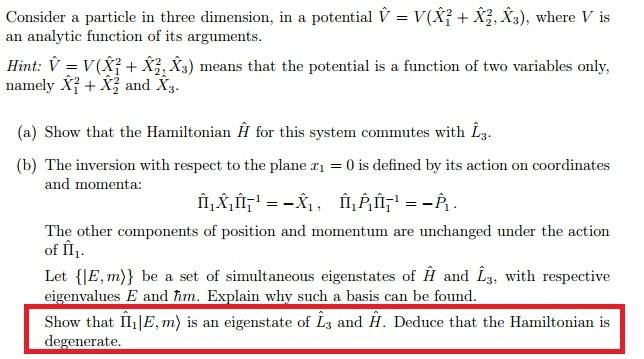# Simultaneous eigenstate of angular momentum and hamiltonian

## Homework StatementThe red box only

## The Attempt at a Solution

I suppose we have to show
L_3 (Π_1) | E,m> = λ (Π_1) | E,m>
and
H (Π_1) | E,m> = μ (Π_1) | E,m>
And I guess there is something to do with the formula given? But they are in x_1 direction so what did they have to do with | E,m> ?
Any hints given will be much appreciated.
Thanksblue_leaf77
Homework Helper
I suppose we have to show
L_3 (Π_1) | E,m> = λ (Π_1) | E,m>
Figure out the behavior of ##L_3## under the transformation induced by ##\Pi_1##.
H (Π_1) | E,m> = μ (Π_1) | E,m>
First show that the Hamiltonian commutes with ##\Pi_1##.

Figure out the behavior of ##L_3## under the transformation induced by ##\Pi_1##.

First show that the Hamiltonian commutes with ##\Pi_1##.

1.I don't know what Π_1 is representing , I mean,like position and momentum operator you have Xψ = xψ , Pψ = -ih d/dx ψ ,but here you have got something like ΠXΠ^-1 = -X which is not the usual form of Kψ = kψ. Then what can I do to compute [ H,Π } ?

2.Since L_3 is acting in the z-direction, Π L_3 ∏^-1 = L_3 ? so Π L_3 = L_3 Π and
L_3 Π | E,m> = Π L_3 | E,m> = m Π | E,m> ?

However ,the question said Π X ∏^-1 is valid for position and momentum operator, but here we are dealing with angular momentum operator?

blue_leaf77
Homework Helper
##\Pi_1## is the parity operator in ##x## direction. It is defined by its action in position space on a wavefunction ##\psi(x_1,x_2,x_3)## by ##\Pi_1 \psi(x_1,x_2,x_3) = \psi(-x_1,x_2,x_3)##. But this is not necessary in the present problem since you are already given by its transformation properties.
here we are dealing with angular momentum operator?
By expanding ##L_3 = x_1 p_2 - x_2 p_1## and using the properties of ##\Pi_1## as given in the question, calculate ##L_3 \Pi_1##.
here you have got something like ΠXΠ^-1 = -X which is not the usual form of Kψ = kψ.
Don't compare them, one is the product between operators and the other one is the application of an operator on a state.
Since L_3 is acting in the z-direction

Last edited:
##\Pi_1## is the parity operator in ##x## direction. It is defined by its action in position space on a wavefunction ##\psi(x_1,x_2,x_3)## by ##\Pi_1 \psi(x_1,x_2,x_3) = \psi(-x_1,x_2,x_3)##. But this is not necessary in the present problem since you are already given by its transformation properties.

By expanding ##L_3 = x_1 p_2 - x_2 p_1## and using the properties of ##\Pi_1## as given in the question, calculate ##L_3 \Pi_1##.

Don't compare them, one is the product between operators and the other one is the application of an operator on a state.

Thanks I got the first part of red box. For the remaining part, I need to show that the Hamiltonian is degenerate. By definition that means E corresponds to more than 1 eigenstate. I am not sure , but from the form of eigenstate | E,m > and the equation H | E,m > = E | E,m > , we see that m does not appear in the eigenvalue of H so I guess m can play a role here? Like E corresponds to | E,1> , | E,0> and | E,-1> ; though I don't know the correct way of saying this.

blue_leaf77
•